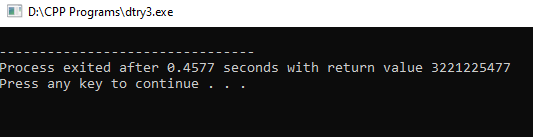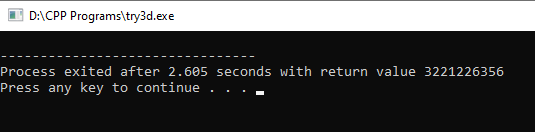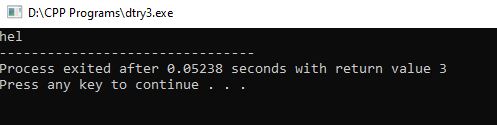×

Search anything:

# Segmentation fault in C and C++

#### C++ Software EngineeringFREE BOOK -> Problems for the day before your Coding Interview (on Amazon)

In this article, we have explored the idea of Segmentation fault in C and C++ along with different possible reasons for Segmentation fault in C and C++.

1. Introduction to Segmentation fault
2. Different Segmentation fault in C and C++

## Introduction to Segmentation fault

There's no much difference between segmentation fault in C and C++.
Let's first understand what is "Segmentation Fault"

Segmentation fault is also called as "access voilation" or in short segfault. This is a failure condition arised due to the hardware with memory protection, OS is notified that the software is trying to access restricted area of a memory. These are due to errors in use of pointer for virtual memory addressing, seen in programming language such as C and C++ which provides low level memory acccess.In other programming languages such as Java and Lisp employ garbage collection to avoid certain class of errors avoiding segmentation faults.

## Different Segmentation fault in C and C++

Following are the different reasons behind Segmentation fault in C and C++:

• Trying to write in read-only portion of memory
• Accessing array out of bounds
• Using variable value as address
• Dereferencing a NULL pointer
• Dereferencing or assigning to an uninitialised pointer
• Dereferencing or assigning to a freed pointer
• Buffer overflow
• Stack overflow

### Now , let's see what causes these segfaults

There can be several different reasons:

• Trying to write in read-only portion of memory

Here let us assume a character pointer to be initialised to some random character . Later if we try to replace it with another character it can't be replaced as it will be assigned as a read only pointer.

Look into the below code snippet

``````// Writing in read only portion
#include<stdio.h>
int main()
{
char *str="a";   //pointer considered as read-only
*str="b";       //Cannot write here
printf("%c",*str); // try to print the value
}
``````

Error:

``````assignment to ‘char’ from ‘char *’ makes integer from pointer without a cast [-Wint-conversion]

Error in line :
5 |     *str="b";       //Cannot write here
``````

You can try printing "*str" using printf for the above code snippet, you will get an error .Later you can try out by commenting the second line i.e. *str="b" you will definetly get the output as the error would have been debugged i.e. we aren't writing into read only portion anymore.

• Accessing array out of bounds

This is a typical case of trying to access an array variable greater than the upper limit. Look into the below code to understand in a better way.

``````// Accessing greater than upper limit
#include<stdio.h>
int main()
{
int arr,i;
for(i=0;i<=6;i++)
{
arr[i]=i;    //error at arr and arr as index ranges between 0 to 4
}
}
``````

Error:

``````*** stack smashing detected ***: terminated
``````
• Using variable value as address

Here we understand the importance of using the "ampersand" symbol in printf and scanf statements which is used to represent address of a variable.
Look into the below code snippet

``````// Usage of & with variable
#include<stdio.h>
int main()
{
int num=0;
scanf("%d",num);    //variable should be passed with & here
}
``````

Error:

``````format ‘%d’ expects argument of type ‘int *’, but argument 2 has type ‘int’ [-Wformat=]

Error at line:
5 |   scanf("%d",num);
``````

Here the address of variable must be passed to get an input i.e. &num as passing of value of the variable will cause a segmentation fault.

• Dereferencing a NULL pointer

Dereferencing generally means manipulating the data of an initialised pointer.Let us assume a pointer is assigned NULL value while initialisation , later when we try to manipulate the value of the NULL pointer.

Look into the below code snippet

``````#include<stdio.h>
int main()
{
int *ptr=NULL;
*ptr=1;          // causing segmenation fault
printf("%d",*ptr);
}
``````

Error:

``````Error: Here,we can't see any error or any warnings but there will be no output for the code
``````

A NULL pointer usually points to an address that's not a part of the processes address space.Hence, dereferencing the Null pointer causes segfault.

• Dereferencing or assigning to an uninitialised pointer

A wild pointer is an uninitialised pointer.
Let us assume a pointer is not assigned with a value while initialisation , later when we try to assign a value to this wild pointer.
Look into the below code snippet

``````#include<stdio.h>
int main()
{
int *ptr;         //wild pointer
*ptr=1;          // causing segmenation fault
printf("%d",*ptr);
}
``````

Error:

``````Error: Here,we can't see any error or any warnings but there will be no output for the code
``````A wild pointer usually points to a random memory address that might or might not be a part of the processes address space. Hence,dereferencing or assigning value to the wild pointer causes segfault.

• Dereferencing or assigning to a freed pointer

A dangling pointer is a freed pointer.
Let us assume a pointer which was allocated some memory space using dynamic memory allocation techniques and was freed using free() function , Now when we try to assign a value to this dangling pointer.
Look into the below code snippet

``````#include<stdio.h>
#inlude<stdlib.h>
int main()
{
int *ptr = malloc(sizeof(int)*10); //dangling pointer
free(ptr);
*ptr=1;          // causing segmenation fault
printf("%d",*ptr);
}
``````• Buffer overflow

Buffer overflow occurs when a program overruns the buffer boundary and overwrites the buffer adjacent memory locations. For example let us consider a character array i.e. string and what happens when the number of characters exceeds the string length.

``````#include<stdio.h>
int main()
{
char s="hello;
printf("%s",s);
}
``````

Output:

``````hel

warning:[Warning] initializer-string for array of chars is too long
``````Value "hel" "lo\0"

Here the char array s was supposed to hold only 3 characters.Hence, the value assigned to s was supposed to store only 3 charcaters but the word "hello" conists 5 characters, overwrites on the adjacent memory address causing segfault.

• Stack overflow

Occurs if the called stack pointer exceeds the stack bounds.The most common cause of stack overflow can be due to excessive or infinite recursion.Recursion is a function in which the function calls itself over and over

Let us look into an example

``````int see()
{
return see();
}
``````

Here, see() is a recursive function calls itself infinite times leading into a segfault.

With this article at OpenGenus, you must have the complete idea of Segmentation fault in C and C++.